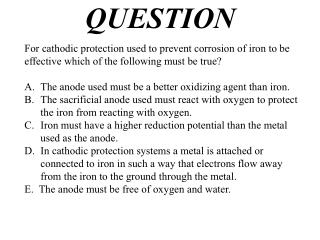Download PresentationQUESTION

# QUESTION - PowerPoint PPT PresentationDownload Presentation## QUESTION

- - - - - - - - - - - - - - - - - - - - - - - - - - - E N D - - - - - - - - - - - - - - - - - - - - - - - - - - -
##### Presentation Transcript

1. QUESTION For cathodic protection used to prevent corrosion of iron to be effective which of the following must be true? The anode used must be a better oxidizing agent than iron. The sacrificial anode used must react with oxygen to protect the iron from reacting with oxygen. Iron must have a higher reduction potential than the metal used as the anode. In cathodic protection systems a metal is attached or connected to iron in such a way that electrons flow away from the iron to the ground through the metal. E. The anode must be free of oxygen and water.

2. ANSWER C. To cathodically protect iron from corrosion is to provide another metal, in contact with the iron sample, to oxidize in place of iron. Thus the other metal sacrifices itself in place of the iron. Only a metal that is a better reducing agent than iron can do this. (A higher reduction potential.)

3. QUESTION • Voltaic cells and electrolytic cells are based on thermodynamic • principles. Which statement about these cells is correct? • The mass of the anode increases in a voltaic cell as • the cell discharges. • B) Reduction occurs at the anode only in the electrolytic • cell. • C) In a voltaic cell, electrons travel from the cathode • to the anode in solution. • D) The free energy change G is greater than zero • for the electrolytic cell.

4. ANSWER • Voltaic cells and electrolytic cells are based on thermodynamic • principles. Which statement about these cells is correct? • The mass of the anode increases in a voltaic cell as • the cell discharges. • B) Reduction occurs at the anode only in the electrolytic • cell. • C) In a voltaic cell, electrons travel from the cathode • to the anode in solution. • D) The free energy change G is greater than zero • for the electrolytic cell.

5. QUESTION How many grams of Cr would plate out from a solution of Cr(NO3)3 when 1.93 × 105 coulombs of charge are passed through the solution? The atomic mass of Cr is 52.0 g/mol, and 1 Faraday is equal to 9.65 × 104 C/mol e−. A) 17.3 g B) 34.7 g C) 52.0 g D) 104 g

6. ANSWER How many grams of Cr would plate out from a solution of Cr(NO3)3 when 1.93 × 105 coulombs of charge are passed through the solution? The atomic mass of Cr is 52.0 g/mol, and 1 Faraday is equal to 9.65 × 104 C/mol e−. A) 17.3 gB) 34.7 g C) 52.0 g D) 104 g Cr3+(aq) + 3 e-Cr(s) 1 mol e- 9.65 x 104 C 1 mol Cr 3 mol e- 52.0 gCr 1 mol Cr 1.93 × 105C xxx =

7. QUESTION

8. B) 2.0 hours Chlorauric acid is one of the few stable gold containing compounds known. Even gold that is dissolved in seawater tends maintain its elemental form. ANSWER - to

9. ANSWER 0.0076 mol e- Calculating charge transfer: AuCl41-(aq) + 3 e-Au(s) + 4 Cl1-(aq) 4.8 x 103 C Calculating the time: 1 mol Au 197 g Au 3 mol e- 1 mol Au 0.50 g Au xx = 0.10 A =0.10 C/s 9.65 x 104 C 1 mol e- Charge (C) = 0.0076 mol e-x = charge (C) Current (C/s) 4.8 x 103 C 0.10 C/s 1 min 60 s 1 hr 60 min Time = = xx = 2 hrs charge (C) time (s) Current (A) =

10. QUESTION

11. A) 2.88 mol ANSWER Cr2O72-(aq) + 12 e-+ 14H+(aq)2 Cr(s) + 7H2O (l) 2H2O(l) 4H+(aq)+ O2(g)+ 4 e- 1 mol Cr 51.996 gCr 6 mol e- 1 mol Cr mol O2 (g) 4 mole- molO2 (g)= 1.00 x 102g Crxxx

12. QUESTION The extraction of aluminum from a mixture of molten Al2O3/Na3AlF6 (cryolite) is superior to the extraction from a solution of aluminum ions in water because… A. carbon dioxide bubbles can continuously provide agitation that effectively stirs the molten solution. B. attempts at reducing Al3+ from water are hampered because Al3+ is a better oxidizing agent than water. C. the process involves lower temperatures (therefore less energy) due to the ease of oxidation of aluminum in the presence of cryolite. D. water is easier to oxidize than aluminum, so it would react first at a lower voltage.

13. ANSWER B. provides the basis for the superiority of the Hall-Heroult process. The E° value for the reduction of Al3+ is 0.83 volts lower than the reduction of water. Therefore, water will be reduced before Al3+ in aqueous solutions. 2011: \$1.15/lb US Patent 400,766; filed July 9, 1886; patented April 2, 1889